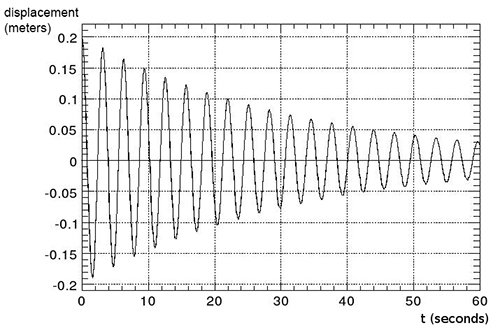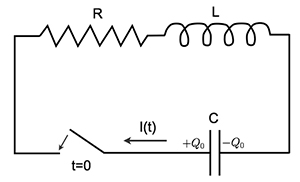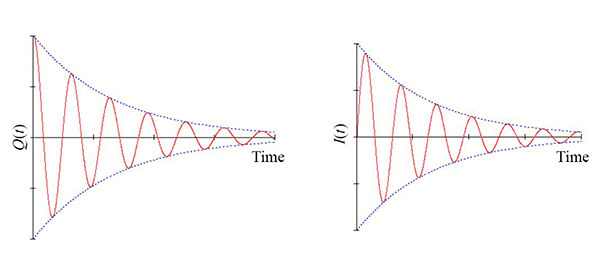RES.8-005 | Fall 2012 | Undergraduate
Vibrations and Waves Problem Solving
Harmonic Oscillators with Damping

## Problems

### Harmonic Oscillators with Damping

#### Problem 1

Using a force of 4 newtons, a damped harmonic oscillator is displaced from equilibrium by 0.2 meters. At t = 0 it is released from rest. The resultant displacement of the oscillator, from the equilibrium position, as a function of time, is shown in the figure below. Estimate, as well as you can using the given information:

1. The mass of the oscillator.
2. The quality factor of the oscillator.The simplest way to do this problem is to obtain the equation of motion from conservation of energy.

Mass and Q of oscillator are approximately 5 kg and 30, respectively.

#### Problem 2The circuit shown above consists of a capacitor ($$C$$), an inductor ($$L$$) and a resistor ($$R$$). Initially the switch is open and the charge ($$Q$$) on the capacitor is $$Q(t \leq 0) =Q_0$$. At $$t = 0$$, the switch is closed.

1. For what values of $$R$$, the initial sign of $$Q(t)$$ will be reversed at some later times?
2. Assuming that the value of $$R$$ satisfies the condition in (1), find $$Q(t)$$ and the current $$I(t)$$ in terms of $$Q_0$$, $$R$$, $$L$$ and $$C$$. Draw qualitative sketches of both $$Q(t)$$ and $$I(t)$$. (Make sure to indicate the behaviour around $$t=0$$).
3. If it takes 10 cycles for the energy in the circuit to decrease to $$1/e$$ times its initial value, find the value of the resistance $$R$$ in terms of $$L$$ and $$C$$.

1.   Initial sign will be reversed only if the circuit is underdamped:

i.e., $$R < 2 \sqrt{\frac{L}{C}}$$

$Q(t) = Q_{0} e^{-\frac{\gamma t}{2}} \left(\cos(\omega’t) + \frac{\gamma}{2 \omega’} \sin(\omega’t)\right)$        and
\begin{align*}
I(t) &= \omega’ Q_{0} e^{-\frac{\gamma t}{2}} \sin(\omega’t) + \frac{\gamma}{2} \frac{Q_{0}\gamma}{2 \omega’} e^{-\frac{\gamma t}{2}} \sin(\omega’t)\\
&= Q_{0}\frac{\omega_{0}^{2}}{\omega’}e^{-\frac{\gamma t}{2}} \sin(\omega’t).
\end{align*}
\begin{equation}
\nonumber{\text{where} \hspace{4mm} \gamma = {\frac{R}{L}} \hspace{1mm} \text{,} \hspace{4mm} \omega_{0}^{2} = {\frac{1}{LC}} \hspace{4mm} \text{and} \hspace{4mm}
\omega’=\sqrt{\omega_{0}^{2}-\frac{\gamma^2}{4}}}
\end{equation}

Below are the sketches of $$Q(t)$$ and $$I(t)$$. Note that $$I(0)=0$$ and both $$Q(t)$$ and $$I(t)$$ oscillate at the same frequency.$R = \dfrac{1}{20\pi}\sqrt{\dfrac{L}{C}}$

« Previous | Next »